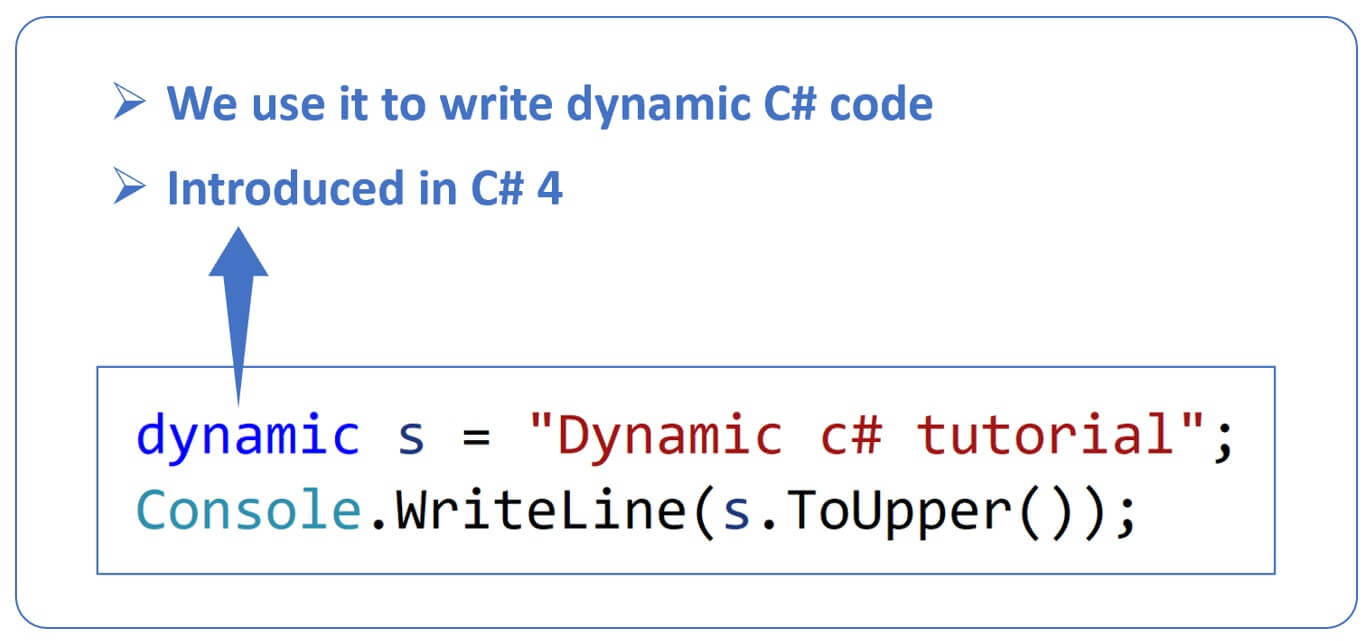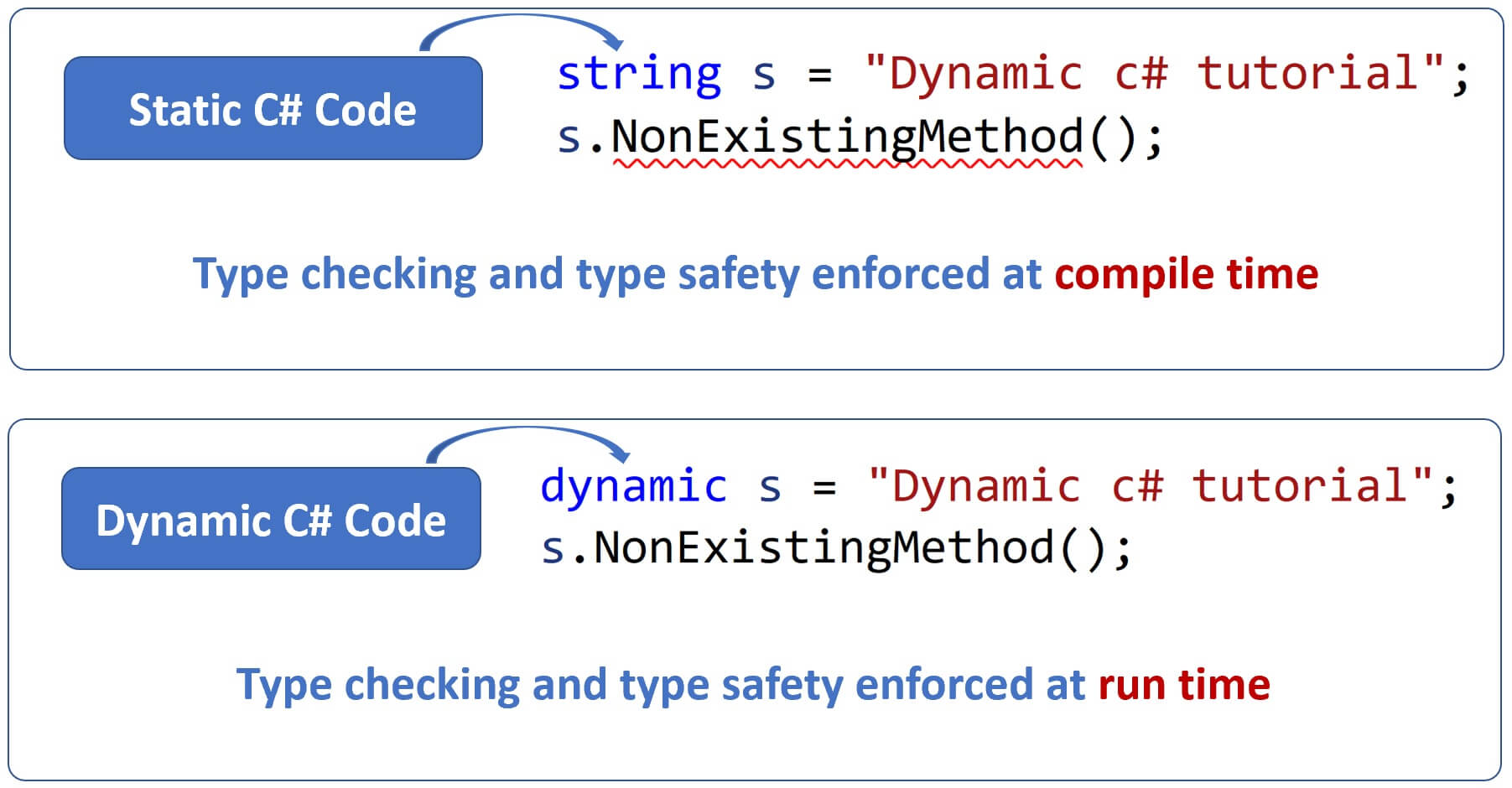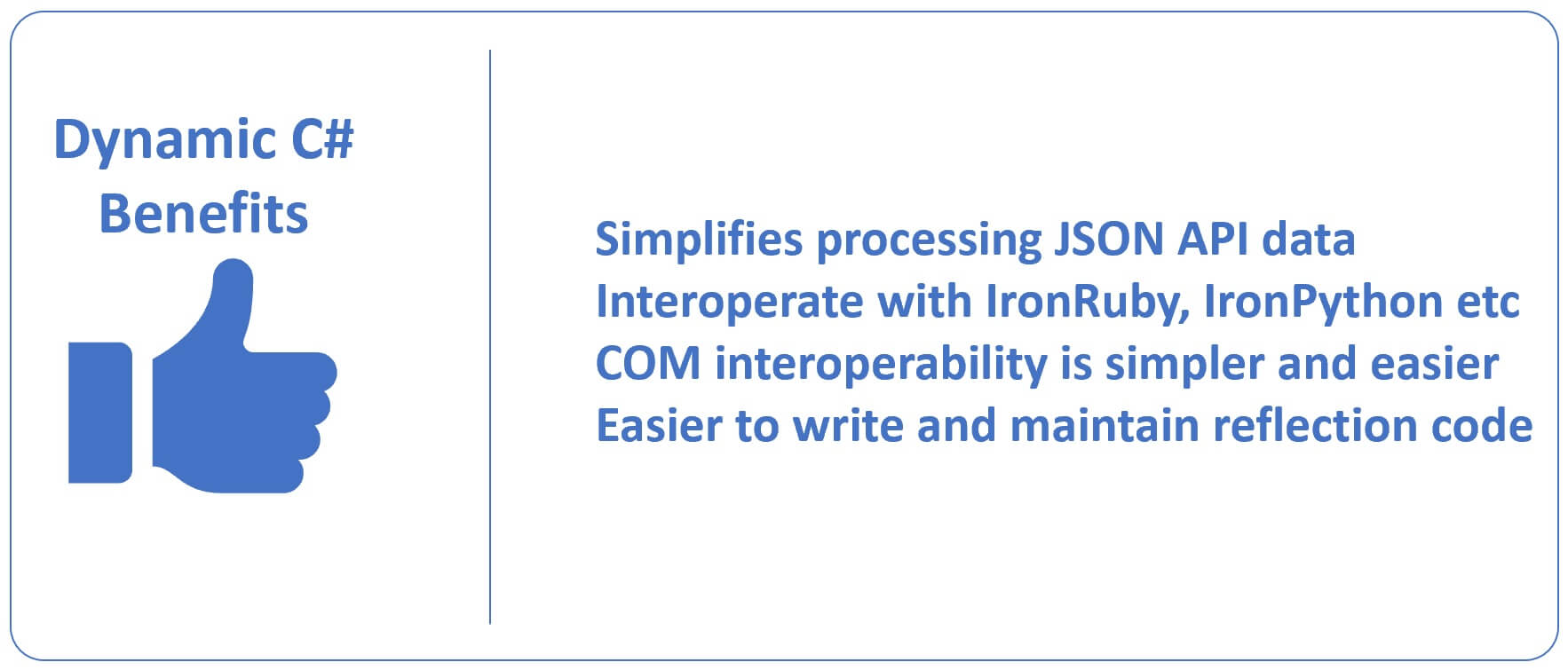# Dynamic C# TutorialIn C# we have several built-in types like

• string
• int
• bool
• DateTime
• etc

All these are static types, meaning `type checking and type safety are enforced at compile-time`. Consider the following example.

``````int i = 100;
long l = i;

Console.WriteLine(\$"int i = {i} & long l = {l}");``````
• long variable `l` is initialized with the value of `int` variable `i`.
• The code complies and runs without issues.
• `int` data type can be converted to a `long` data type without any data loss.
• The compiler allows this implicit conversion.

The following code will not compile. Fails with the error - Cannot implicitly convert type 'long' to 'int'.

``````long l = 100;
int i = l;``````

An implicit conversion is not allowed in this case, because `long` data type has much bigger range than `int` data type and there is a scope for data loss.

We can use an explicit cast if we really need to covert a `long` type to `int` as shown below. The compiler allows this, because we are doing the conversion explicitly i.e we are making the decision consciously, we know converting long to int can result in data loss, but in this case the long variable has a value of 100 which can be safely converted to int without data loss.

``````long l = 100;
int i = (int)l;``````

Consider the following code. The compiler knows the variable `s` is of type `string` and it has `ToUpper()` instance method. So this code complies and runs without any issues.

``````string s = "Dynamic c# tutorial";
Console.WriteLine(s.ToUpper());``````

This code on the other hand will not compile because the compiler knows `string` type does not have `NonExistingMethod()`.

``````string s = "Dynamic c# tutorial";
s.NonExistingMethod();``````

This compile time checking of the code is called static binding and it's a good thing because we are able to catch errors at compile time instead of runtime.

## C# dynamic type

This new type `dynamic` is introduced in C# 4 and as the name implies we use it to write dynamic c# code. This code compiles and runs without issues.The code below compiles but at runtime an exception is thrown beacuse the string type does not have `NonExistingMethod()`.

``````dynamic s = "Dynamic c# tutorial";
s.NonExistingMethod();``````

So, the important point to keep in mind is, even with dynamic c#, type checking and type saftey is enforced. The only difference is it is enforced at runtime instead of compile time. With static c# code, type checking and type saftey are enforced at compile time and with dynamic c# code they are enforced at runtime.The following code prints the actual type

``````dynamic dynamicString = "Dynamic c# tutorial";
Console.WriteLine(\$"Type is {dynamicString.GetType()} & value = {dynamicString}");

dynamic dynamicInt = 1000;
Console.WriteLine(\$"Type is {dynamicInt.GetType()} & value = {dynamicInt}");``````

Output

``````Type is System.String & value = Dynamic c# tutorial
Type is System.Int32 & value = 1000``````

## Conversion from static types to dynamic and vice versaConversion from static types like int, double, float etc to dynamic and vice versa does not require an explicit cast. These conversions are done implicitly.

``````// Convert from int to dynamic
int i1 = 100;
dynamic d1 = i1;

Console.WriteLine(\$"i1 = {i1} & d1 = {d1}");

// Convert from dynamic to int
dynamic d2 = 200;
int i2 = d2;

Console.WriteLine(\$"i2 = {i2} & d2 = {d2}");``````

This is true even with complex types like Customer, Employee etc.

## Convert complex Employee type to dynamic

``````class Program
{
static void Main(string[] args)
{
Employee e1 = new Employee()
{
FirstName = "Pragim",
LastName = "Tech"
};

// Employee type to dynamic conversion
dynamic dynamicEmployee = e1;

Console.WriteLine(\$"First Name = {dynamicEmployee.FirstName}");
Console.WriteLine(\$"Last Name = {dynamicEmployee.LastName}");
}
}

class Employee
{
public string FirstName { get; set; }
public string LastName { get; set; }
}``````

## Convert dynamic to Employee type

``````class Program
{
static void Main(string[] args)
{
dynamic dynamicEmployee = new Employee()
{
FirstName = "Pragim",
LastName = "Tech"
};

// dynamic to Employee type conversion
Employee e = dynamicEmployee;

Console.WriteLine(\$"First Name = {e.FirstName}");
Console.WriteLine(\$"Last Name = {e.LastName}");
}
}

class Employee
{
public string FirstName { get; set; }
public string LastName { get; set; }
}``````

## Dynamic implicit conversions

When there is no potential for data loss, c# allows certain data type conversions to happen implicitly, for example from a double to int, long to int etc. Double and long have much bigger range than int, so converting to int will not result in any sort of data loss, hence the conversion happens implicitly. This is true both with static and dynamic c#.

``````int i1 = 100;
double d = i1;
Console.WriteLine(\$"int i1 = {i1} & dobule d = {d}");

int i2 = 200;
long l = i2;
Console.WriteLine(\$"int i2 = {i2} & long l = {l}");

// int to dynamic to double - implicit conversion
int i1 = 100;
dynamic dynInt = i1;
double d = dynInt;
Console.WriteLine(\$"int i1 = {i1} & Dyn Int = {dynInt} & double d = {d}");

// int to dynamic to long - implicit conversion
int i2 = 200;
dynamic dynInt2 = i2;
long l = dynInt2;
Console.WriteLine(\$"int i2 = {i2} & Dyn Int = {dynInt2} & long l = {l}");``````

## Explicit conversions

Converting large data types to smaller data types is not allowed implicitly because there is a potential for data loss. You can use an explicit cast though. Again, this is true for both static and dynamic c#.

``````// Convert double to int. Fails to compile, an explicit cast is required
// Error : Cannot implicitly convert type double to int
double d1 = 1000;
// int i1 = d1;

// Explicit cast from double to int
int i1 = (int)d1;
Console.WriteLine(\$"d1 = {d1} & i1 = {i1}");

// Even with dynamic c# an explicit cast is required when
// converting larger data types like double to int
double d = 1000;
dynamic dynDouble = d;
int i1 = (int)dynDouble;

Console.WriteLine(\$"double d = {d} & dynDouble = {dynDouble} && int i1 = {i1}");``````

## Why use dynamic C#

By looking at the examples we discussed so far you might be thinking, why do we need dynamic c#, what benefits it provides? Well, dynamic C# has several benefits.### Simplifies processing JSON API data

When an API returns JSON data, we usually create another strongly types class in our application and map JSON data. However, in scenarios where we do not want to create yet another model class but still want to be able to consume and process JSON data, we can make use of dynamic C#. We will see an example of this in our upcoming videos.

### Interoperate with other languages like IronRuby or IronPython

Dynamic c# makes it possible to interoperate with other programming languages like IronRuby or IronPython. If you are wondering why do we need to interoperate with other programming languages? Well, to use features of other languages that C# doesn't support.

### COM interoperability is simpler and easier

With the help of dynamic C# we can perform COM interop even if we don't have reference to the interop assemblies, so COM interoperability is simpler and easier.

### Easier to write and maintain reflection code

With dynamic c# it's easier to write reflection code which inturn makes the code more readable and maintainable.

#### Invoking an instance method using reflection

Consider the following simple `Calculator` class.

``````public class Calculator
{
public int Add(int fn, int sn)
{
return fn + sn;
}
}``````

The following is the code required to invoke `Add` method using reflection.

``````Calculator calculator = new Calculator();

System.Reflection.BindingFlags.InvokeMethod,
null,
calculator,
new object[] { 10, 20 });

Console.WriteLine(\$"Sum = {result}");``````
• We have lot of code just to call a method.
• The cod is complex and difficult to understand.

The above `reflection` code is rewritten using `dynamic c#`. This is simpler, cleaner and easier to understand.

``````dynamic calculator = new Calculator();
Dot net provides a built-in dynamic type called `ExpandoObject`. Syncfusion Blazor components support dynamic c# and ExpandoObject. We will discuss ExpandoObject and using it with Syncfusion blazor components in our upcoming videos.